Functions and CALL Routines

# CONSTANT Function

Computes machine and mathematical constants.
 Category: Mathematical

## Syntax

 CONSTANT(constant<, parameter>)

### Arguments

constant

is a character constant, variable, or expression that identifies the constant to be returned. Valid constants are as follows:

Description Constant
The natural base
`'E'`
Euler constant
`'EULER'`
Pi
`'PI'`
Exact integer
`'EXACTINT' <,nbytes>`
The largest double-precision number
`'BIG'`
The log with respect to base of BIG
`'LOGBIG' <,base>`
The square root of BIG
`'SQRTBIG'`
The smallest double-precision number
`'SMALL'`
The log with respect to base of SMALL
`'LOGSMALL' <,base>`
The square root of SMALL
`'SQRTSMALL'`
Machine precision constant
`'MACEPS'`
The log with respect to base of MACEPS
`'LOGMACEPS' <,base>`
The square root of MACEPS
`'SQRTMACEPS'`

parameter

is an optional numeric parameter. Some of the constants specified in constant have an optional argument that alters the functionality of the CONSTANT function.

CAUTION:
In some operating environments, the run-time library might have limitations that prevent the use of the full range of floating-point numbers that the hardware provides.

In such cases, the CONSTANT function attempts to return values that are compatible with the limitations of the run-time library.

For example, if the run-time library cannot compute EXP(LOG(CONSTANT('BIG'))) , then CONSTANT('LOGBIG') will not return the same value as LOG(CONSTANT('BIG')) , but will return a value such that EXP(CONSTANT('LOGBIG')) can be computed.## Syntax

 CONSTANT('E')

The natural base is described by the following equation:
## Syntax

 CONSTANT('EULER')

Euler's constant is described by the following equation:## Syntax

 CONSTANT('PI')

Pi is the ratio between the circumference and the diameter of a circle. Many expressions exist for computing this constant. One such expression for the series is described by the following equation:
## Syntax

 CONSTANT('EXACTINT' <, nbytes>)

where

nbytes

is a numeric value that is the number of bytes.

 Range: 2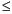nbytes8 Default: 8

The exact integer is the largest integer k such that all integers less than or equal to k in absolute value have an exact representation in a SAS numeric variable of length nbytes. This information can be useful to know before you trim a SAS numeric variable from the default 8 bytes of storage to a lower number of bytes to save storage.

## Syntax

 CONSTANT('BIG')

This case returns the largest double-precision floating-point number (8-bytes) that is representable on your computer.

## Syntax

 CONSTANT('LOGBIG' <, base>)

where

base

is a numeric value that is the base of the logarithm.

 Restriction: The base that you specify must be greater than the value of 1+SQRTMACEPS. Default: the natural base, E.

This case returns the logarithm with respect to base of the largest double-precision floating-point number (8-bytes) that is representable on your computer.

It is safe to exponentiate the given base raised to a power less than or equal to CONSTANT('LOGBIG', base) by using the power operation (**) without causing any overflows.

It is safe to exponentiate any floating-point number less than or equal to CONSTANT('LOGBIG') by using the exponential function, EXP, without causing any overflows.

## Syntax

 CONSTANT('SQRTBIG')

This case returns the square root of the largest double-precision floating-point number (8-bytes) that is representable on your computer.

It is safe to square any floating-point number less than or equal to CONSTANT('SQRTBIG') without causing any overflows.

## Syntax

 CONSTANT('SMALL')

This case returns the smallest double-precision floating-point number (8-bytes) that is representable on your computer.

## Syntax

 CONSTANT('LOGSMALL' <, base>)

where

base

is a numeric value that is the base of the logarithm.

 Restriction: The base that you specify must be greater than the value of 1+SQRTMACEPS. Default: the natural base, E.

This case returns the logarithm with respect to base of the smallest double-precision floating-point number (8-bytes) that is representable on your computer.

It is safe to exponentiate the given base raised to a power greater than or equal to CONSTANT('LOGSMALL', base) by using the power operation (**) without causing any underflows or 0.

It is safe to exponentiate any floating-point number greater than or equal to CONSTANT('LOGSMALL') by using the exponential function, EXP, without causing any underflows or 0.

## Syntax

 CONSTANT('SQRTSMALL')

This case returns the square root of the smallest double-precision floating-point number (8-bytes) that is representable on the computer.

It is safe to square any floating-point number greater than or equal to CONSTANT('SQRTBIG') without causing any underflows or 0.

## Syntax

 CONSTANT('MACEPS')

This case returns the smallest double-precision floating-point number (8-bytes)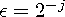for some integer j, such that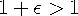.

This constant is important in finite precision computations.

## Syntax

 CONSTANT('LOGMACEPS' <, base>)

where

base

is a numeric value that is the base of the logarithm.

 Restriction: The base that you specify must be greater than the value of 1+SQRTMACEPS. Default: the natural base, E.

This case returns the logarithm with respect to base of CONSTANT('MACEPS').

## Syntax

 CONSTANT('SQRTMACEPS')

This case returns the square root of CONSTANT('MACEPS').Previous Page | Next Page | Top of Page